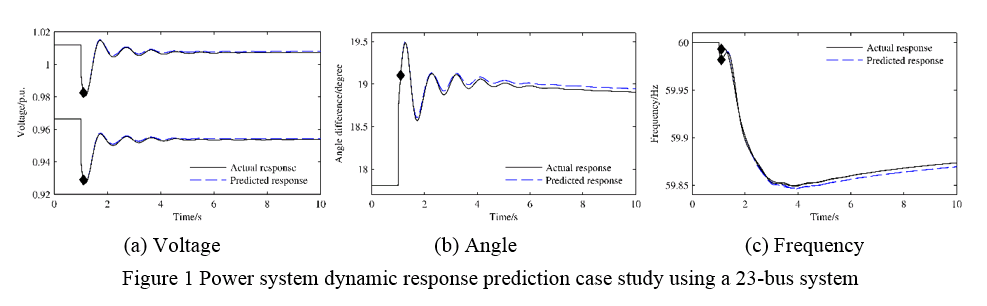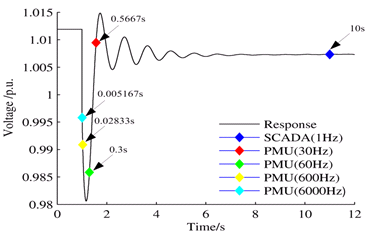## EECS Home »

Power IT Lab » Research

## Dynamic Response Prediction Using Phasor Data ModelFigure 2 Prediction start time as a function of PMU data rate

The ability to predict power system dynamics could provide system operators with look-ahead capabilities and allow control actions to take place in advance. Traditionally, simulation is the only predictive tool, but it has many limitations such as model inaccuracy and simulation speed for large scale systems. This work takes advantage of the power grid linearity property and constructs a linear-structured model using pure measurements to predict a large-scale power grids dynamic behavior following a disturbance. A linear multivariate autoregressive (MAR) model derived from measurement data was developed. The prediction process includes training the MAR model with measurement data to obtain a prediction model that can model power system dynamics and also predict the system response recursively with a few initial data points following a certain disturbance [1-2]. Figure 1 shows a case study using a 23-bus system. In this case study, the linear MAR model was developed with 15 s-long data from a previous disturbance. This model was then used to predict the system response following another disturbance in this system. With an order of 13, it can be seen that the predicted responses by a linear MAR model are very accurate when compared with actual responses. It was observed that if the PMU measurement data rate could be increased further into the future, this prediction method has the potential to start as early as several milliseconds after the disturbance (as shown in Figure 2).

References

 C. Li, Y. Liu, K. Sun, Y. Liu, and N. Bhatt, "Measurement based Power System Dynamics Prediction with Multivariate Autoregressive Model," in Proc. IEEE PES Transm. and Distrib. Conf. and Expo., Chicago, IL, 2014.

 C. Li, J. Chai, Y. Liu, N. Bhatt, A. D. Rosso, and E. Farantatos, "Power System Dynamics Prediction with Measurement-Based AutoRegressive Model", 2014 CIGRE US Conference, Houston, TX, US, 2014.